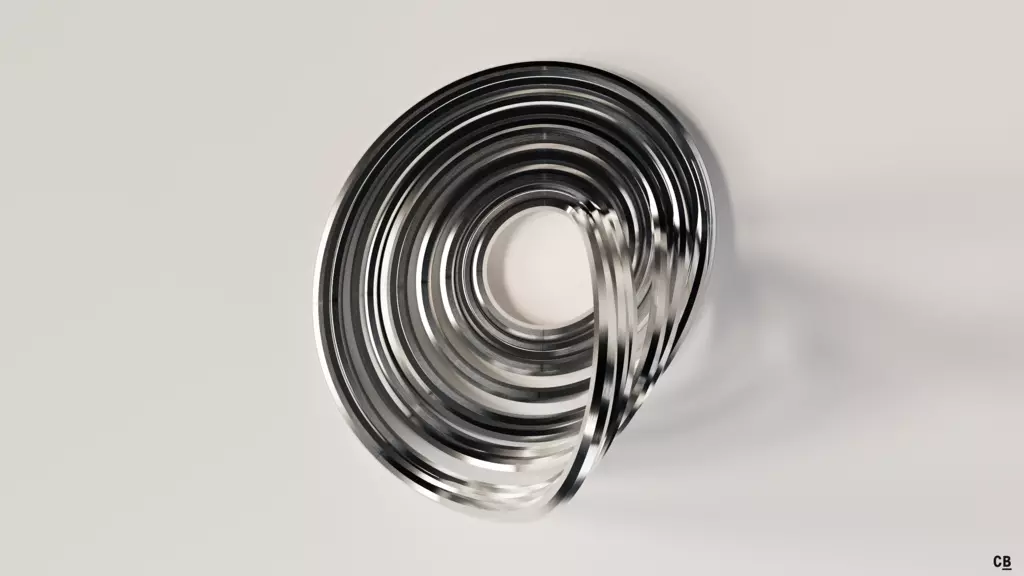# Roessler Attractor

After the Lorenz attractor was found and intensively studied, the German biochemist Otto Eberhard Rössler simplified the model in 1976 and discovered a system that contains only one wing1.

The resulting attractor has interesting topological properties as part of it is similar to a Möbius strip.

## Renders

Differential system:

$\dot{x} = -y - z$ $\dot{y} = x + a\, y$ $\dot{z} = b + z\, (x - c)$

Constants:

$a = 0.2$ $b = 0.2$ $c = 5.7$1. O.E. Rössler, 1976. "An equation for continuous chaos". Phys. Let. A. 57(05). doi:10.1016/0375-9601(76)90101-8

🕸 💍